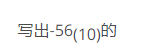HR
【简答题】八位二进制原码：__八位二进制补码：__手机使用分享复制链接新浪微博分享QQ微信扫一扫反馈A.

B.

A.

B.

A.

B.

A.

B.

UPS开机后，面板无显示，UPS不工作，可能原因有交流电输入保险烧毁造成交流无输入、蓄电池电压检测回路问题等。
A.

B.

A.

B.

UPS在有交流电输入时输出正常，无交流输入时蜂鸣器长鸣无输出，可能为蓄电池或逆变器部分故障。
A.

B.

UPS蓄电池电压偏低，开机充电十小时电压仍偏低，则判断蓄电池损坏，断开蓄电池则充电电路输入输出正常。
A.

B.

UPS处于逆变状态时，逆变器有输出但电压偏低，同时变压器发出噪音，可能原因为推挽电路的输出不对称。
A.

B.

A.

B.

A.

B.

A.

B.

A.

B.

A.

B.

A.

B.

A.

B.

A.

B.

A.

B.

A.

B.

A.

B.

A.

B.

A.

B.

A.

B.

A.

B.

A.

B.

A.

B.

A.

B.

A.

B.

A.

B.

A.

B.

A.

B.

A.

B.

A.

B.

A.

B.

A.

B.

A.

B.

A.

B.

A.

B.

A.

B.

A.

B.

A.

B.

A.

B.

A.

B.

A.

B.

A.

B.

A.

B.

A.

B.

A.

B.

A.

B.

A.

B.

A.

B.

A.

B.

A.

B.

A.

B.

A.

B.

A.

B.

A.

B.

A.

B.

A.

B.

A.

B.

A.

B.

A.

B.

A.

B.

A.

B.

RCS-9785C的GPS插件不仅可以接收GPS天线的信号，而且通过光纤输入接口可以接收来自另一台时钟源的(____)。
A.
IRIG-B时间码
B.

C.

D.

RCS-9785D装置一般具有(____)个GPS插件。
A.
1
B.
2
C.
3
D.
4

A.

B.

C.

D.

A.

B.

C.

D.

A.
B.
C.

D.

A.

B.

C.

D.

A.

B.

C.

D.

1PPS 脉冲信号是(____)。
A.

B.

C.

D.

1PPS 脉冲信号是(____)对时方式。
A.
B.

C.
B码
D.

1PPM 脉冲信号是(____)。
A.

B.

C.

D.

1PPM脉冲信号是(____)对时方式。
A.
B.

C.
B码
D.

1PPH脉冲信号是(____)对时方式。
A.
B.

C.

D.

IRIG-B时间码为美国靶场仪器组提出国际通用时间格式码，并分成A、B、C、D、E、G、H几种，电力系统的时间同步对时中最为常用的是IRIG-B，传输介质可用(____)。
A.

B.

C.

D.

IRIG-B（AC）时间码是用IRIG-B（DC）时间码对1kHz正弦波进行幅度调制形成的时间码信号，幅值大的对应高电平，幅值小的对应低电平，调制比(____) ~6:1连续可调，典型调制比3:1。
A.
2:1
B.
3:1
C.
4:1
D.
5:1

A.
RS-242
B.
RS-485
C.

D.

A.
30S
B.
60S
C.
90S
D.
120S

A.

B.
6个1
C.
6个8
D.

A.

B.

C.

D.

A.
6个1
B.
C.
6个8
D.

A.
10
B.
20
C.
30
D.
40

A.
C
B.
D
C.
E
D.
F

A.

B.
U盘
C.

D.

A.

B.

C.

D.

A.

B.

C.

D.

A.

B.

C.

D.

A.
1
B.
2
C.
5
D.
10

A.

B.

C.

D.

A.

B.

C.

D.

A.

B.

C.

D.

A.

B.

C.

D.

A.
1，3线对是一个绕对；2，6线对是一个绕对；4，5线对是一个绕对；7，8线对是一个绕对
B.
1，2线对是一个绕对；3，5线对是一个绕对；4，6线对是一个绕对；7，8线对是一个绕对
C.
1，2线对是一个绕对；7，6线对是一个绕对；4，5线对是一个绕对；3，8线对是一个绕对
D.
1，2线对是一个绕对；3，6线对是一个绕对；4，5线对是一个绕对；7，8线对是一个绕对

A.

B.

C.

D.

IP地址由4个字节(____)位构成，用来表示网络地址及主机地址。
A.
8
B.
16
C.
32
D.
64

A.

B.

C.

D.

A.

B.

C.

D.

A.

B.

C.

D.143%